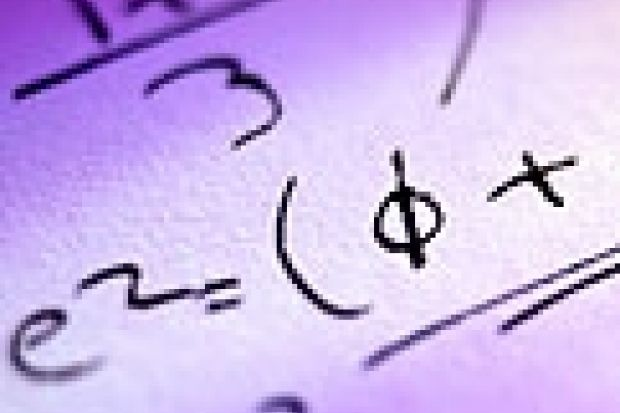# Look at that figure

January 26, 2001Why are monstrous moonshine theorems more interesting than other concepts in mathematics? Simon Colton sums up the allure of the pure

In many ways, pure mathematics is as much an art form as a science. Few of its theorems are going to save lives or help build better mobile phones, but, like Picasso masterpieces, they bring enjoyment and interest to thousands of people. In the mathematical reasoning group at Edinburgh University, we are looking at why some pure mathematics results are more interesting than others. Understanding "interestingness" is a key factor in our project to build mathematical theories by computer. Our program, called HR after the number theory pioneers Godfrey Hardy and Srinivasa Ramanujan, starts with simple concepts such as addition and subtraction, then invents new concepts, finds examples and suggests and proves theorems.

While the program works in many different areas, it has been most successful in number theory. For instance, HR invented the concept of refactorable numbers, where the number of divisors is itself a divisor. For example, 8 is a refactorable number because it has 4 divisors (1, 2, 4 and 8 all divide 8 with no remainders) and 4 itself is a divisor. What is more, HR found there is something unusual about odd refactorables, such as 1, 9 and 225. They are all square numbers: 1 is 1 squared, 9 is 3 squared and 225 is 15 squared. It turned out that refactorable numbers had been investigated a decade before, but HR was possibly first to notice that odd refactorables are squares - something we eventually proved ourselves. While not exactly rocket science, this kind of fact makes these numbers more interesting.

In such programs, where new concepts are built from the most interesting old ones, it is essential to spot the best. HR provides some general indicators of interestingness, but the user must decide which are important for the task in hand. Too few examples usually make a concept dull, though this is no guarantee. We can also look at its definition, as simple concepts are often more interesting than complicated ones. For a truly interesting, easy-to-understand concept, consider the prime numbers 2, 3, 5, 7 and 11, which have exactly two divisors. We know some very important results involving prime numbers, not least that every number bigger than 1 can be written as some primes multiplied together. For example, 105 = 3 x 5 x 7. There are also many unsolved questions about primes, adding to their appeal.

The mathematician John Conway, on being asked what makes a good theory, said: "It must be outrageous." It is true that when two concepts that should have nothing in common appear to be related, this relationship can generate massive interest. In fact, the Fields medal - the mathematics equivalent of a Nobel prize - was recently awarded to Richard Borcherds of Cambridge University for proving some theorems that relate concepts from two very different areas of mathematics, dubbed the "monstrous moonshine theorems". The importance of a theorem becomes clear as people use it in different ways, such as taking short cuts in calculations or proving many related theorems.

Equipped with notions of interestingness, computer programs are starting to make discoveries in pure mathematics. Siemion Fajtlowicz's Graffiti program has worked in graph theory for more than a decade, and there are more than 60 papers by influential mathematicians about its theorems. Another program recently discovered a remarkable new formula for pi that the mathematicians had missed. Our HR program has added 20 new concepts to the 60,000 contained in the Encyclopedia of Integer Sequences . It also supplied some interesting theorems about each concept, as only interesting concepts are allowed into the encyclopedia. HR discovered, for example, that if you take a number such as 25, and the sum of its divisors (1+5+25=31) is a prime as here, then the number of divisors you have just added up will also be prime.

Next March, the third annual Symposium on Artificial Intelligence and Creativity in Arts and Science will be held in York, during one of the United Kingdom's largest AI events ( www.aisb.org.uk/2001convention &lt;/a&gt; ). Creating paintings, music, jokes, poems and theorems by computer is a growing area in AI. We hope programs such as HR and Graffiti will eventually be found inside computer algebra systems (major pieces of software able to perform very complicated mathematical calculations).

One day, when someone investigates, for example, refactorable numbers, in addition to calculating thousands of examples, the program will say: "Hey, have you noticed that all odd refactorables are square?" This may not arouse the same excitement as a Picasso masterpiece, but to us it is just as interesting.

Simon Colton is a research associate in the division of informatics at the University of Edinburgh. http:///www.dai.ed.ac.uk/simonco

&lt;/a&gt;

## Please login or register to read this article.

### Register to continue

Get a month's unlimited access to THE content online. Just register and complete your career summary.

Registration is free and only takes a moment. Once registered you can read a total of 3 articles each month, plus:

• Sign up for the editor's highlights
• Receive World University Rankings news first
• Get job alerts, shortlist jobs and save job searches
• Participate in reader discussions and post comments

## Have your say

Log in or register to post comments